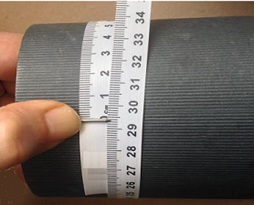©Ms. Garcia Math Name: ID: Quiz: Grade: Find diameter given circumference [d = C/π ] π (Pi) = 3.14 3.1 3 ? Complete this table (round the answer to the nearest tenth)Radius (cm) Diameter (cm) Circumference (cm) 1) 2) 3) 4) Divide the circumference by Pi to find the diameter of a circle. 5) 6)